Courses

# Chapter Notes - Algebra Class 6 Notes | EduRev

## Mathematics (Maths) Class 6

Created by: Praveen Kumar

## Class 6 : Chapter Notes - Algebra Class 6 Notes | EduRev

The document Chapter Notes - Algebra Class 6 Notes | EduRev is a part of the Class 6 Course Mathematics (Maths) Class 6.
All you need of Class 6 at this link: Class 6

11. Algebra

Variables: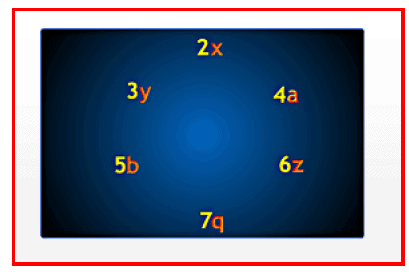An unknown quantity can be represented by a variable.

An unknown quantity can be represented by a variable. Usually, a variable is any letter from the English alphabet that represents an unknown quantity. The relation between the unknown quantity and other quantities can be expressed with the help of the variable. The value of the variable varies with the given condition on the variable. A quantity whose value does not vary is called a constant. An expression consisting of variables, constants and mathematical operators is called an algebraic expression.

Mathematical operations such as addition, subtraction, multiplication and division can be easily performed on variables. We can use variables to form expressions based on patterns.

The following are some branches of mathematics:

• The branch of mathematics where letters are used along with numbers is called algebra.
•  The branch of mathematics that deals with numbers, operations on numbers and properties of numbers is called arithmetic.
•  The branch of mathematics that deals with the figures and shapes is called geometry.

Page 49

Use of Variables: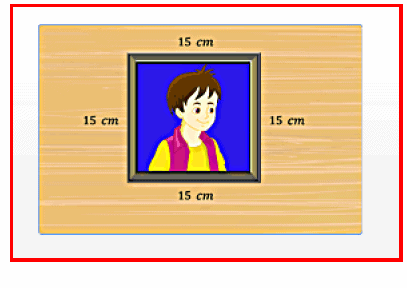Variables are used to frame rules to find the perimeter of a polygon.

Variables are used to frame rules to find the perimeter of a polygon. The perimeter of a polygon can be obtained by adding the lengths of its sides. The following are simple rules to frame the perimeter of geometrical figures using variables.

 If the length of the side is denoted by variable's', then the perimeter of a squar e is equal to 4s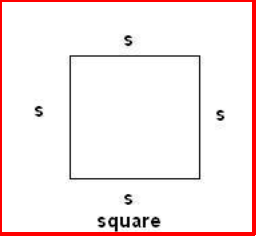‘l’ and its breadth variable 4s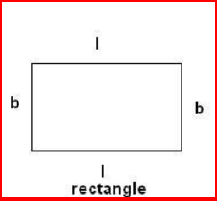If the lengths ol the sides ol a triangle are denoted by x,y and z then the perimeter of the triangle is squal to x + y + z.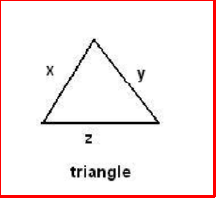Page 50

The following are some simple rules for the properties of numbers using variables.

This property states that two numbers can be added in any order. If a and b represent any two numbers, then a+b=b+a

Commutative property of multiplication:

This property states that two numbers can be multiplied in any order. If a and b represent any two numbers, then a*b=b*a

This property states that three numbers can be added in any order. If a,b and c represent any three numbers, then  (a + b) + c = a + (b + c)

Associative property of multiplication:

This property states that three numbers can be multiplied in any order. If  a,b and c represent any three numbers, then  (a x b) x c = a x (b x c)

Distributive property of multiplication over addition:

This property states that if a,b and c represent any three numbers, then ax (b + c) = a x b + a x c

EquationsA mathematical statement that indicates that the value of the LHS is equal to the value of the RHS is called an equation.

Page 51

A mathematical statement that indicates that the value of the LHS is equal to the value of the RHS is called an equation.
An equation puts a condition on the variable.
The value for which the equation is satisfied is the solution of the equation.

Eg: The equation n/3 = 15 is satisfied for n = 45.
The value of the variable in an equation that satisfies the equation, or makes its LHS equal to its RHS, is the solution.
An equation can contain numbers and variables.

Eg: a - 2 = 30, 72÷9 = 8
An equation is said to be algebraic equation if it consists of a variable.

20x=400

A single variable equation will have a unique solution.

Eg; 15n = 225
An equation that does not have any variable is called a numerical or an arithmetic equation.

Eg: 17 X 2 = 34
Different numerical values for the variable are substituted in an algebraic equation, and the solution is obtained by using a method called the trial and error method. If there is no sign of equality between the LHS and the RHS, then it is not an equation.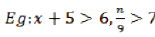are not equations.

,

,

,

,

,

,

,

,

,

,

,

,

,

,

,

,

,

,

,

,

,

;